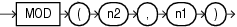## MOD

SyntaxDescription of the illustration mod.eps

Purpose

`MOD` returns the remainder of `n2` divided by `n1`. Returns `n2` if `n1` is 0.

This function takes as arguments any numeric data type or any nonnumeric data type that can be implicitly converted to a numeric data type. Oracle determines the argument with the highest numeric precedence, implicitly converts the remaining arguments to that data type, and returns that data type.

Table 2-8 for more information on implicit conversion and for information on numeric precedence

Examples

The following example returns the remainder of 11 divided by 4:

```SELECT MOD(11,4) "Modulus"
FROM DUAL;

Modulus
----------
3
```

This function behaves differently from the classical mathematical modulus function, if the product of `n1` and `n2` is negative. The classical modulus can be expressed using the `MOD` function with this formula:

```n2 - n1 * FLOOR(n2/n1)
```

The following table illustrates the difference between the `MOD` function and the classical modulus:

n2 n1 MOD(n2,n1) Classical Modulus

`11`

`4`

`3`

`3`

`11`

`-4`

`3`

`-1`

`-11`

`4`

`-3`

`1`

`-11`

`-4`

`-3`

`-3`

FLOOR and REMAINDER, which is similar to `MOD`, but uses `ROUND` in its formula instead of `FLOOR`